Plots of Rhat statistics, ratios of effective sample size to total sample size, and autocorrelation of MCMC draws. See the Plot Descriptions section, below, for details. For models fit using the No-U-Turn-Sampler, see also MCMC-nuts for additional MCMC diagnostic plots.

mcmc_rhat(rhat, ..., size = NULL)

mcmc_rhat_hist(rhat, ..., binwidth = NULL, breaks = NULL)

mcmc_rhat_data(rhat, ...)

mcmc_neff(ratio, ..., size = NULL)

mcmc_neff_hist(ratio, ..., binwidth = NULL, breaks = NULL)

mcmc_neff_data(ratio, ...)

mcmc_acf(
x,
pars = character(),
regex_pars = character(),
...,
facet_args = list(),
lags = 20,
size = NULL
)

mcmc_acf_bar(
x,
pars = character(),
regex_pars = character(),
...,
facet_args = list(),
lags = 20
)

## Arguments

rhat

A vector of R-hat estimates.

...

Currently ignored.

size

Optional values to override ggplot2::geom_point()'s default size (for mcmc_rhat(), mcmc_neff()) or ggplot2::geom_line()'s default line width (for mcmc_acf()).

binwidth

Passed to ggplot2::geom_histogram() to override the default binwidth.

breaks

Passed to ggplot2::geom_histogram() as an alternative to binwidth.

ratio

A vector of ratios of effective sample size estimates to total sample size. See neff_ratio().

x

An object containing MCMC draws:

• A 3-D array, matrix, list of matrices, or data frame. The MCMC-overview page provides details on how to specify each these.

• A draws object from the posterior package (e.g., draws_array, draws_rvars, etc.).

• An object with an as.array() method that returns the same kind of 3-D array described on the MCMC-overview page.

pars

An optional character vector of parameter names. If neither pars nor regex_pars is specified then the default is to use all parameters. As of version 1.7.0, bayesplot also supports 'tidy' parameter selection by specifying pars = vars(...), where ... is specified the same way as in dplyr::select(...) and similar functions. Examples of using pars in this way can be found on the Tidy parameter selection page.

regex_pars

An optional regular expression to use for parameter selection. Can be specified instead of pars or in addition to pars. When using pars for tidy parameter selection, the regex_pars argument is ignored since select helpers perform a similar function.

facet_args

A named list of arguments (other than facets) passed to ggplot2::facet_wrap() or ggplot2::facet_grid() to control faceting. Note: if scales is not included in facet_args then bayesplot may use scales="free" as the default (depending on the plot) instead of the ggplot2 default of scales="fixed".

lags

The number of lags to show in the autocorrelation plot.

## Value

The plotting functions return a ggplot object that can be further customized using the ggplot2 package. The functions with suffix _data() return the data that would have been drawn by the plotting function.

## Plot Descriptions

mcmc_rhat(), mcmc_rhat_hist()

Rhat values as either points or a histogram. Values are colored using different shades (lighter is better). The chosen thresholds are somewhat arbitrary, but can be useful guidelines in practice.

• light: below 1.05 (good)

• mid: between 1.05 and 1.1 (ok)

• dark: above 1.1 (too high)

mcmc_neff(), mcmc_neff_hist()

Ratios of effective sample size to total sample size as either points or a histogram. Values are colored using different shades (lighter is better). The chosen thresholds are somewhat arbitrary, but can be useful guidelines in practice.

• light: between 0.5 and 1 (high)

• mid: between 0.1 and 0.5 (good)

• dark: below 0.1 (low)

mcmc_acf(), mcmc_acf_bar()

Grid of autocorrelation plots by chain and parameter. The lags argument gives the maximum number of lags at which to calculate the autocorrelation function. mcmc_acf() is a line plot whereas mcmc_acf_bar() is a barplot.

Other MCMC: MCMC-combos, MCMC-distributions, MCMC-intervals, MCMC-nuts, MCMC-overview, MCMC-parcoord, MCMC-recover, MCMC-scatterplots, MCMC-traces

## Examples

# autocorrelation
x <- example_mcmc_draws()
dim(x)
#>  250   4   4
dimnames(x)
#> $Iteration #> NULL #> #>$Chain
#>  "chain:1" "chain:2" "chain:3" "chain:4"
#>
#> \$Parameter
#>  "alpha"   "sigma"   "beta" "beta"
#>

color_scheme_set("green")
mcmc_acf(x, pars = c("alpha", "beta"))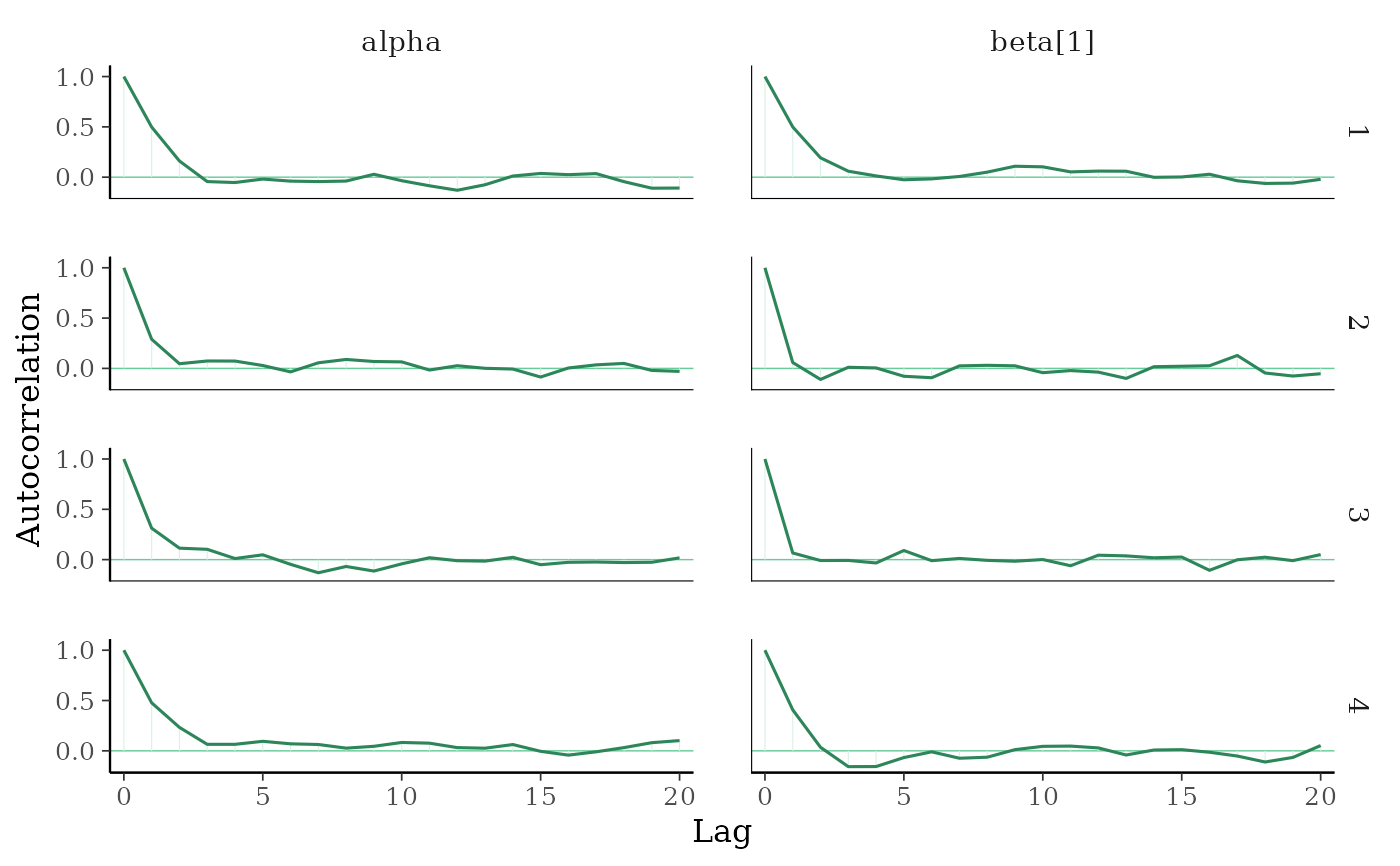# \donttest{
color_scheme_set("pink")
(p <- mcmc_acf_bar(x, pars = c("alpha", "beta")))# add horiztonal dashed line at 0.5
p + hline_at(0.5, linetype = 2, size = 0.15, color = "gray")# }

# fake rhat values to use for demonstration
rhat <- c(runif(100, 1, 1.15))
mcmc_rhat_hist(rhat)
#> stat_bin() using bins = 30. Pick better value with binwidth.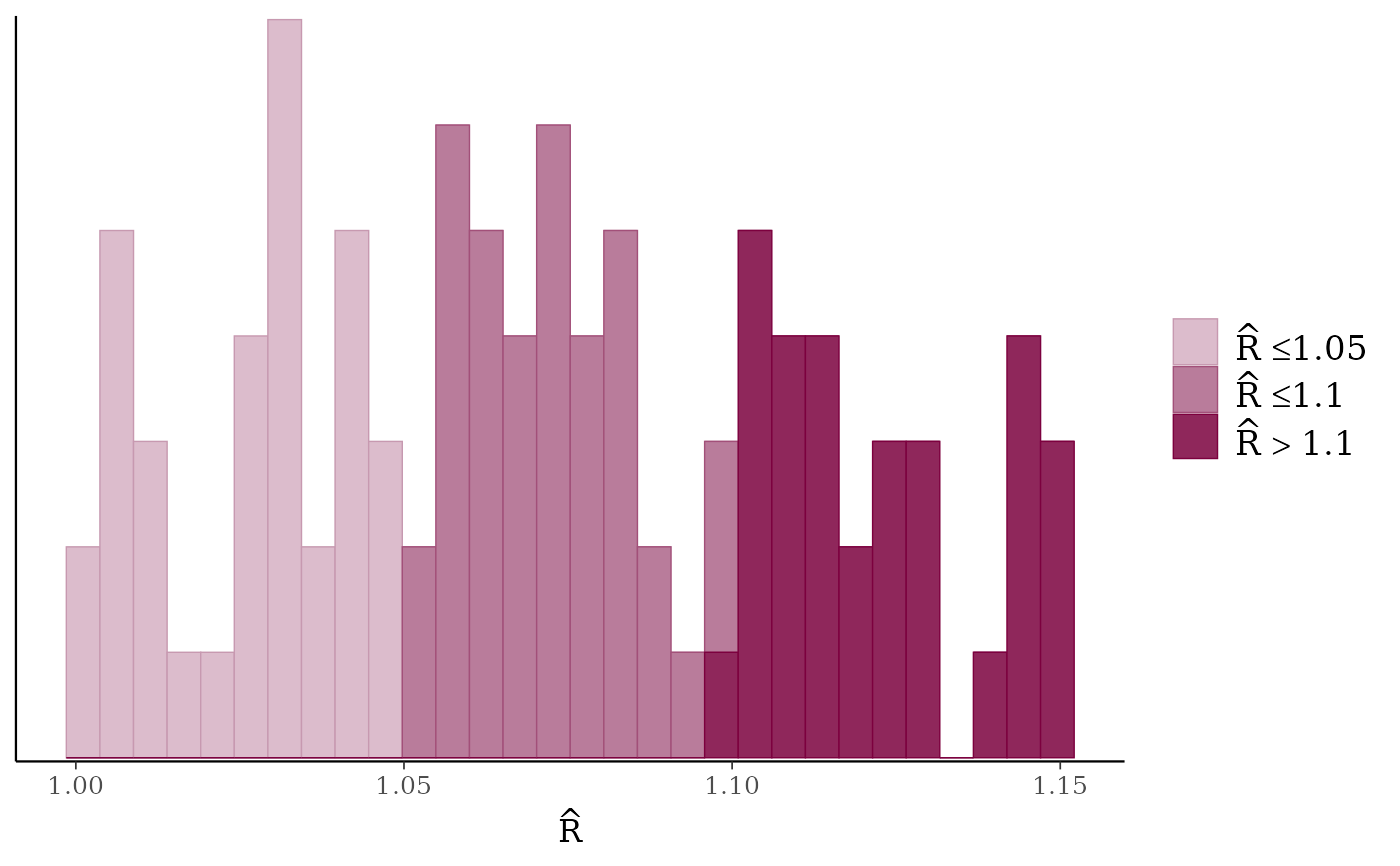mcmc_rhat(rhat)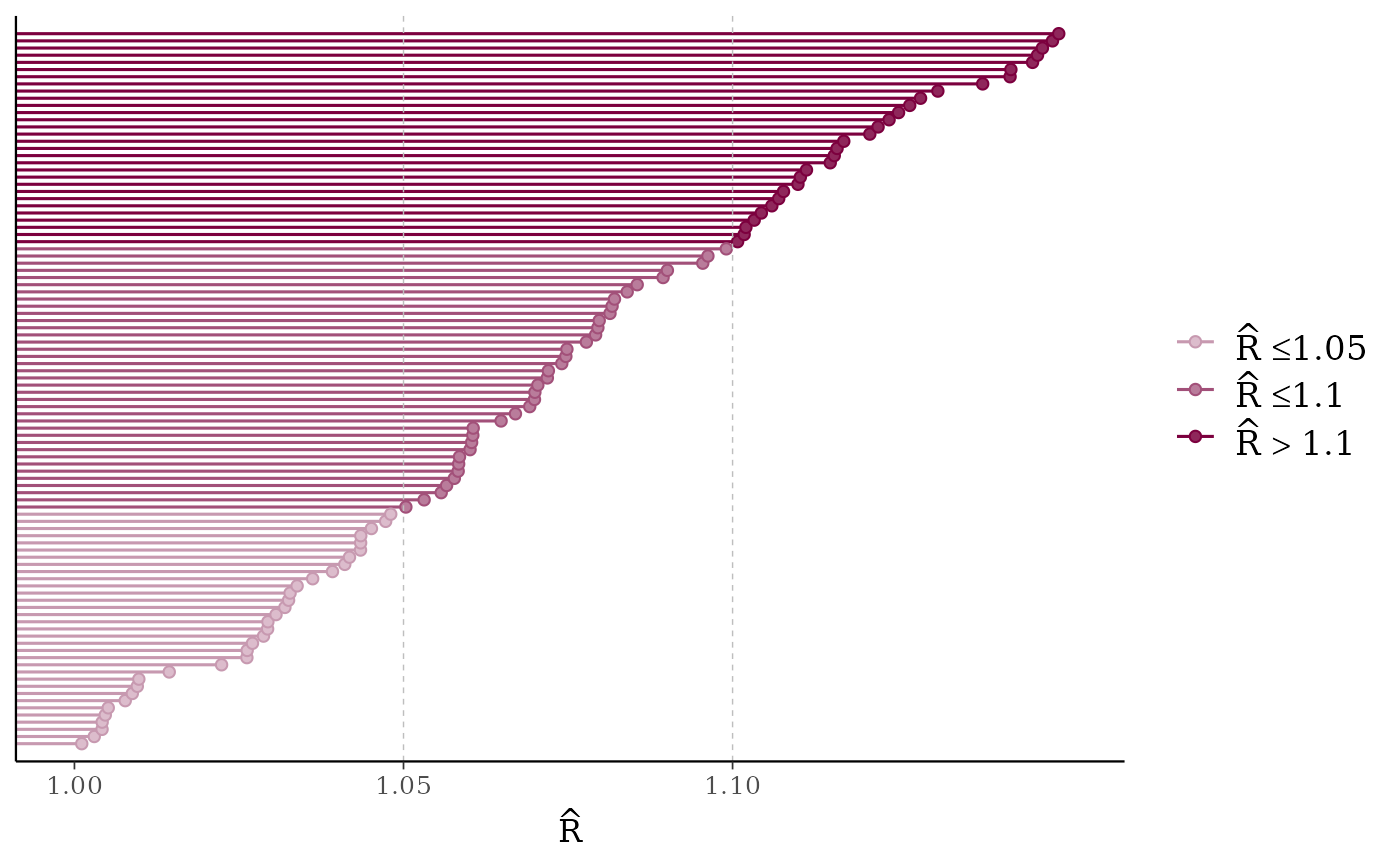# lollipops
color_scheme_set("purple")
mcmc_rhat(rhat[1:10], size = 5)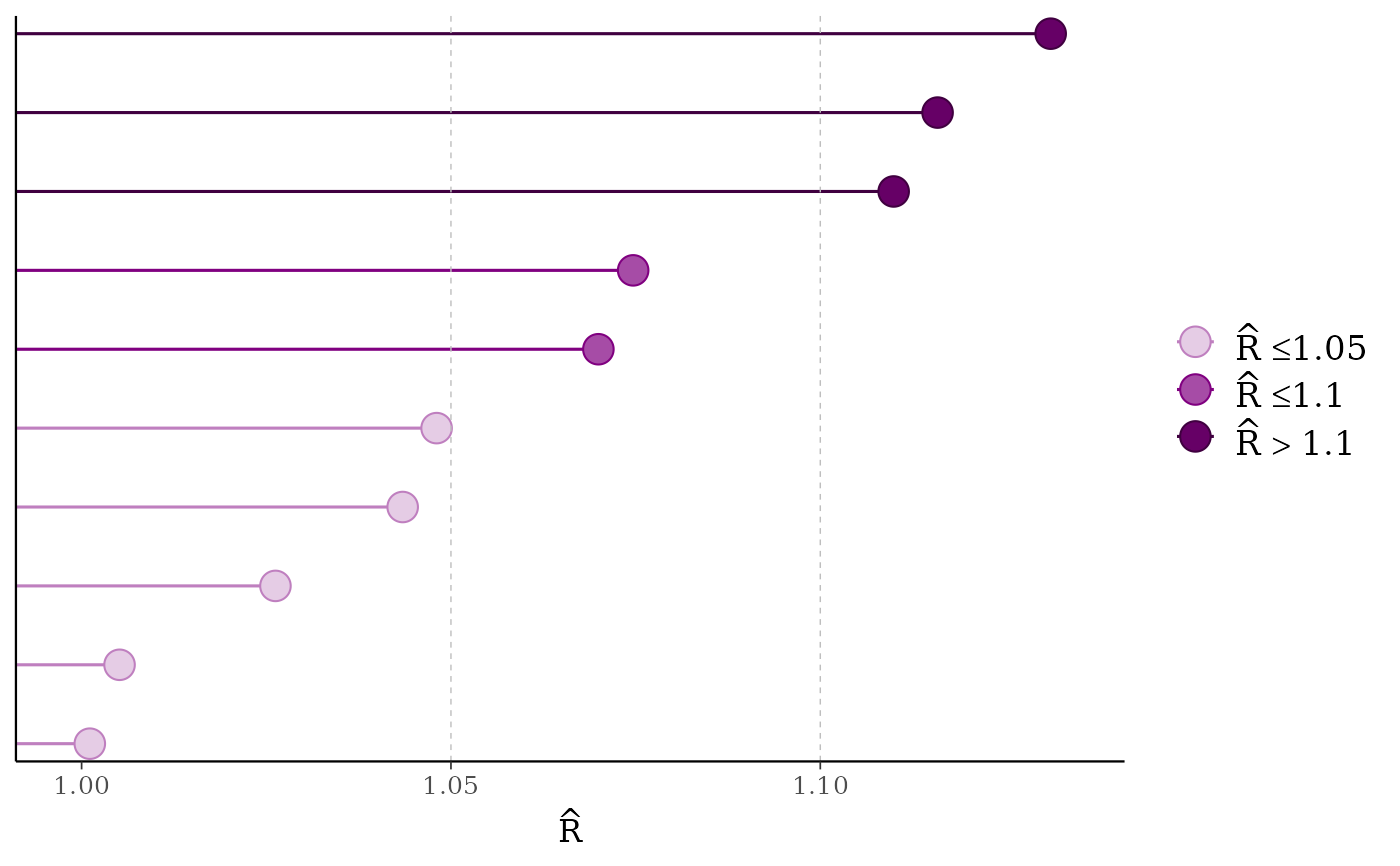color_scheme_set("blue")
mcmc_rhat(runif(1000, 1, 1.07))mcmc_rhat(runif(1000, 1, 1.3)) + legend_move("top") # add legend above plot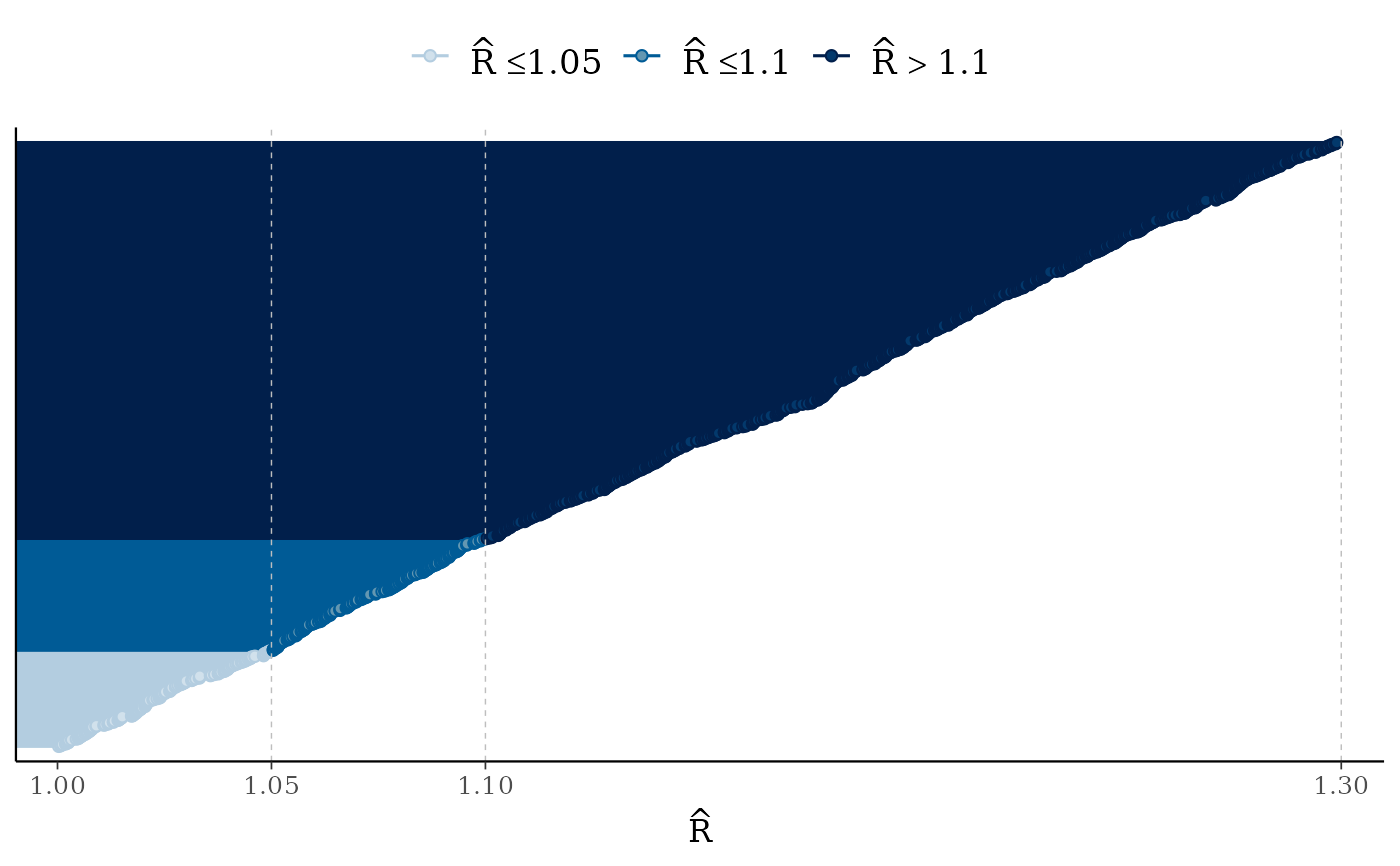# fake neff ratio values to use for demonstration
ratio <- c(runif(100, 0, 1))
mcmc_neff_hist(ratio)
#> stat_bin() using bins = 30. Pick better value with binwidth.mcmc_neff(ratio)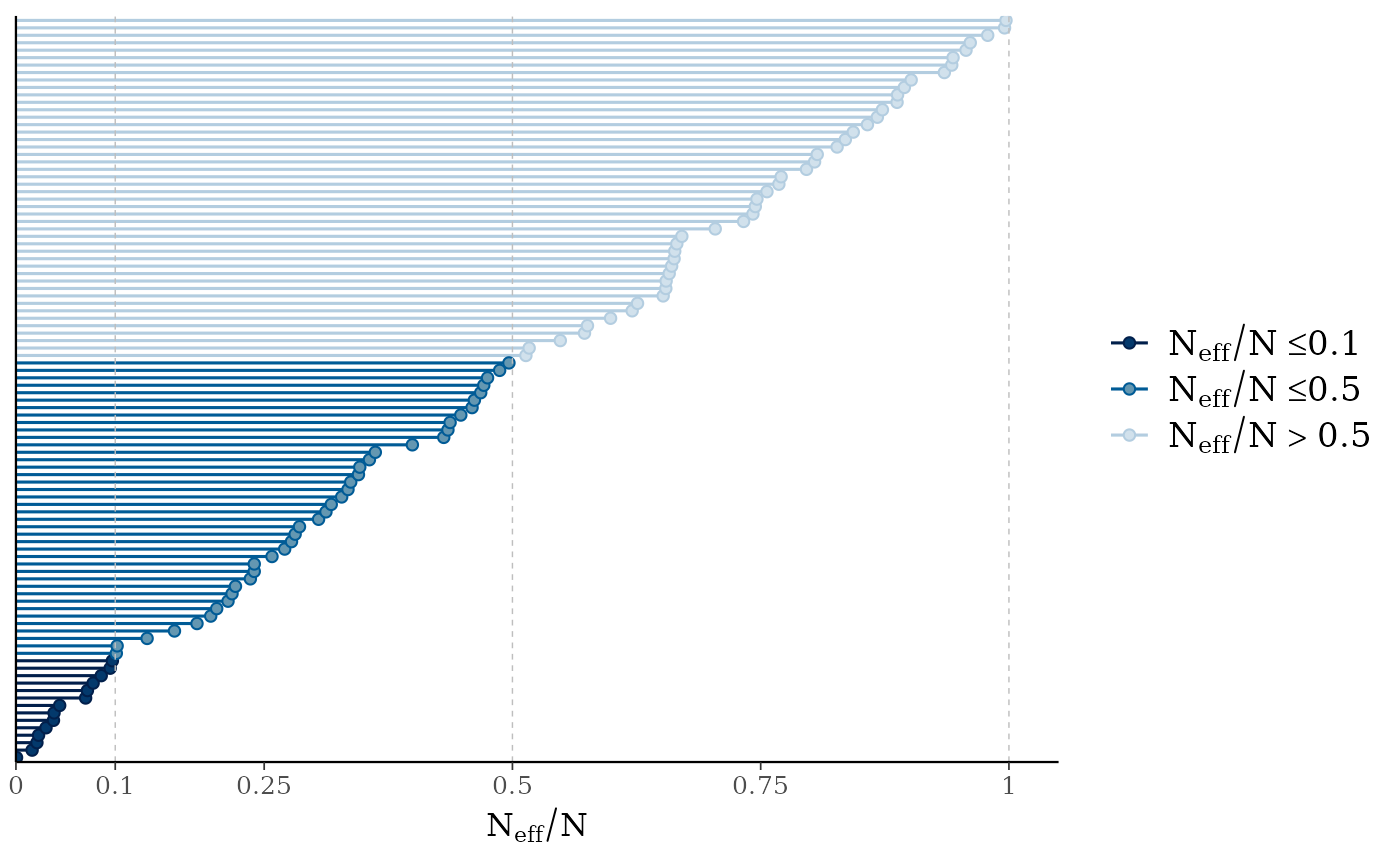# \dontrun{
# Example using rstanarm model (requires rstanarm package)
library(rstanarm)
#> This is rstanarm version 2.21.3
#> - See https://mc-stan.org/rstanarm/articles/priors for changes to default priors!
#> - Default priors may change, so it's safest to specify priors, even if equivalent to the defaults.
#> - For execution on a local, multicore CPU with excess RAM we recommend calling
#>   options(mc.cores = parallel::detectCores())

# intentionally use small 'iter' so there are some
# problems with rhat and neff for demonstration
fit <- stan_glm(mpg ~ ., data = mtcars, iter = 50, refresh = 0)
#> Warning: The largest R-hat is 1.36, indicating chains have not mixed.
#> Running the chains for more iterations may help. See
#> https://mc-stan.org/misc/warnings.html#r-hat
#> Warning: Bulk Effective Samples Size (ESS) is too low, indicating posterior means and medians may be unreliable.
#> Running the chains for more iterations may help. See
#> https://mc-stan.org/misc/warnings.html#bulk-ess
#> Warning: Tail Effective Samples Size (ESS) is too low, indicating posterior variances and tail quantiles may be unreliable.
#> Running the chains for more iterations may help. See
#> https://mc-stan.org/misc/warnings.html#tail-ess
#> Warning: Markov chains did not converge! Do not analyze results!
rhats <- rhat(fit)
ratios <- neff_ratio(fit)
mcmc_rhat(rhats)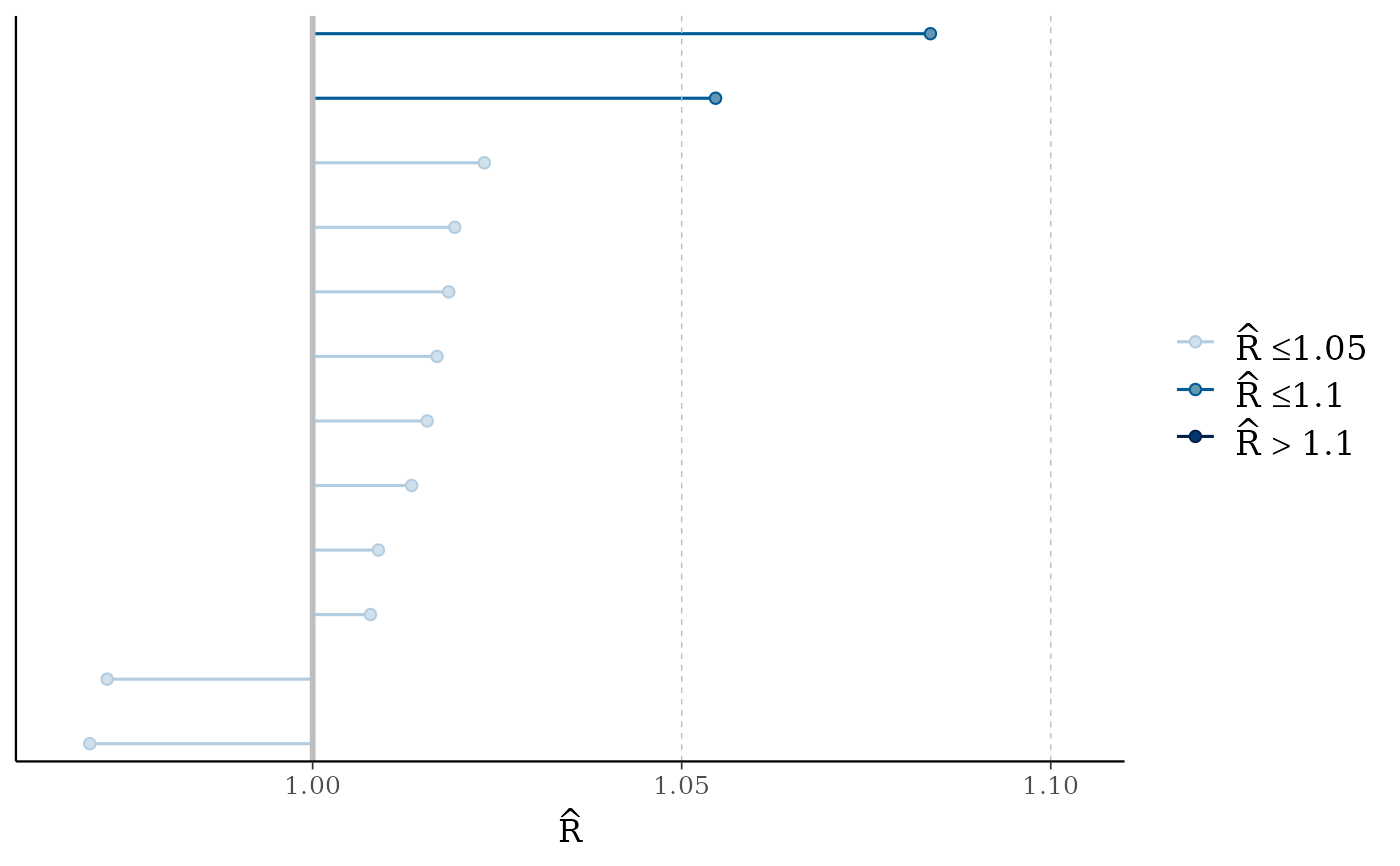mcmc_neff(ratios, size = 3)# there's a small enough number of parameters in the
# model that we can display their names on the y-axis
mcmc_neff(ratios) + yaxis_text(hjust = 1)# can also look at autocorrelation
draws <- as.array(fit)
mcmc_acf(draws, pars = c("wt", "cyl"), lags = 10)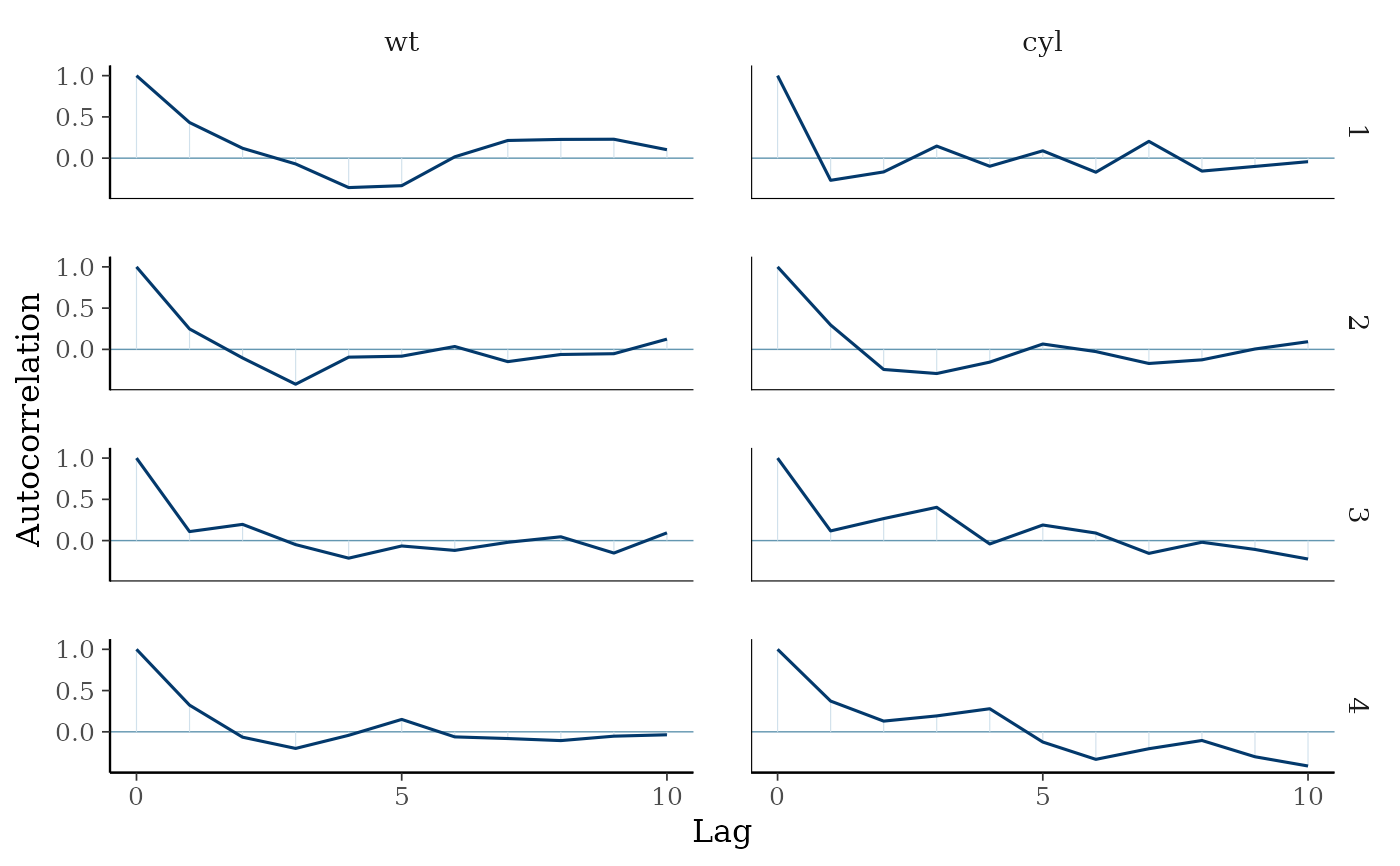# increase number of iterations and plots look much better
fit2 <- update(fit, iter = 500)
#> Warning: Bulk Effective Samples Size (ESS) is too low, indicating posterior means and medians may be unreliable.
#> Running the chains for more iterations may help. See
#> https://mc-stan.org/misc/warnings.html#bulk-ess
mcmc_rhat(rhat(fit2))mcmc_neff(neff_ratio(fit2))mcmc_acf(as.array(fit2), pars = c("wt", "cyl"), lags = 10)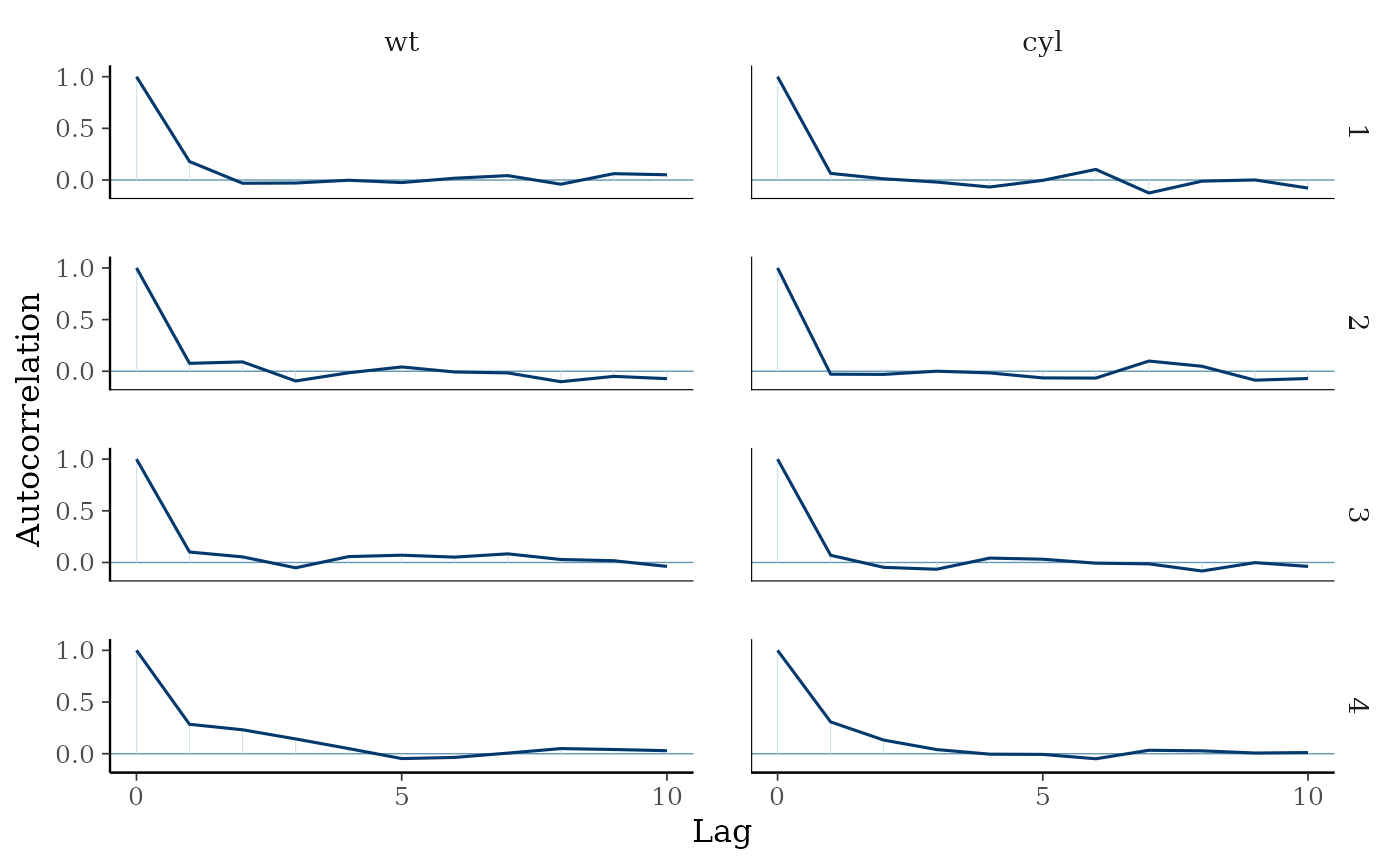# }